# Precalculus : Determine the Direction in Which a Parabola Opens

## Example Questions

### Example Question #1 : Determine The Direction In Which A Parabola Opens

Determine the direction in which the following parabola opens, if the y-axis is vertical and the x-axis is horizontal: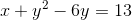Down

Up

Left

Along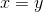Right

Left

Explanation:

In order to determine which direction the parabola opens, we must first put the equation in standard form, which can be expressed in one of the following two ways: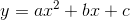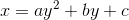If the equation is foras in the first above, the parabola opens up ifis positive and down ifis negative. If the equation is foras in the second above, the parabola opens right ifis positive and left ifis negative. Rearranging our equation, we get: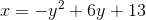We can see that our equation is for, which means the parabola will open either left or right. The sign of the first term is negative, so this parabola will open to the left.

### Example Question #2 : Determine The Direction In Which A Parabola Opens

Which direction does the parabola open?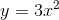Upwards

Downwards

Rightwards

Leftwards

Upwards

Explanation:

For the function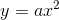The parabola opens upwards if a>0

and downards for a<0

Because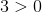The parabola opens upwards.

### Example Question #3 : Determine The Direction In Which A Parabola Opens

Determine the direction which the parabola opens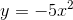.

Upwards

Leftwards

Downwards

Rightwards

Downwards

Explanation:

For the functionThe parabola opens upwards if a>0

and downards for a<0

Because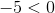The parabola opens downwards.

### Example Question #4 : Determine The Direction In Which A Parabola Opens

Determine what direction the following parabola opens: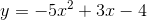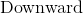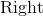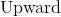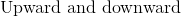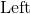Explanation:

The standard form for a parabola is in the form: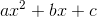The coefficient of theterm determines whether if the parabola opens upward or downward.  Since theterm in the functionis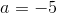, the parabola will open downward.

### Example Question #5 : Determine The Direction In Which A Parabola Opens

Determine what direction will the following function open: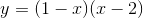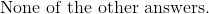Explanation:

Use the FOIL method to determinein its standard form for parabolas, which is.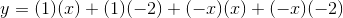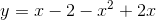Regroup the terms.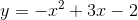Since the coefficient of theterm is negative, the parabola will open downward.

### Example Question #6 : Determine The Direction In Which A Parabola Opens

If a parabola has vertex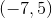and focus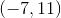, which direction will it open?

right

left

we would need to know the directrix to determine the parabola's direction

down

up

up

Explanation:

The focus is above the vertex, which means that the parabola will open up

### Example Question #7 : Determine The Direction In Which A Parabola Opens

Determine the direction in which the parabola will open.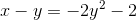Up

Down

Left

The graph is a straight line.

Right

Left

Explanation:

In order to determine which way this parabola, group the variables in one side of the equation.  Addon both sides of the equation to isolate.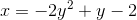Because the equation is in terms of, the parabola will either open left or right.  Notice that the coefficient of the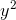term is negative.

The parabola will open to the left.

### Example Question #8 : Determine The Direction In Which A Parabola Opens

Determine whether the following parabola opens up or down and state how you know.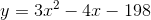Up, because the squared term is positive.

Up, because the linear term is negative.

Down, because the constant term is negative.

Down, because the linear term is negative.

Up, because the squared term is positive.

Explanation:

Determine whether the following parabola opens up or down and state how you know.To determine the direction a parabola opens, we only need to worry about the squared term.

In this case, it is positive, so the parabola opens upward.

The linear term is negative, so the parabola will be to the right of the y-axis.

The constant term is negative, so the parabola will be located below the x-axis.

### Example Question #9 : Determine The Direction In Which A Parabola Opens

Determine which direction the equation opens: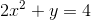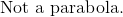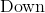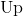Explanation:

In order to determine how the parabola will open, we will need to rewrite the equation in standard form.

Write the standard form for parabolas.Subtract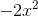from both sides of the equation.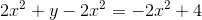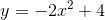Since the coefficient of theis negative, and the equation is in terms of, the parabola will open downward.

The answer is:### Example Question #10 : Determine The Direction In Which A Parabola Opens

Which is the equation for a parabola that opens down?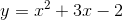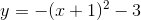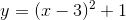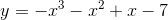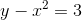The answer isbecause it is the only degree-2 polynomial with a negative leading coefficient.